Friday, October 22, 2021
Home > Latest Announcement > NSEJS 2018-19 Question Paper With Answer Keys And Solutions

# NSEJS 2018-19 Question Paper With Answer Keys And Solutions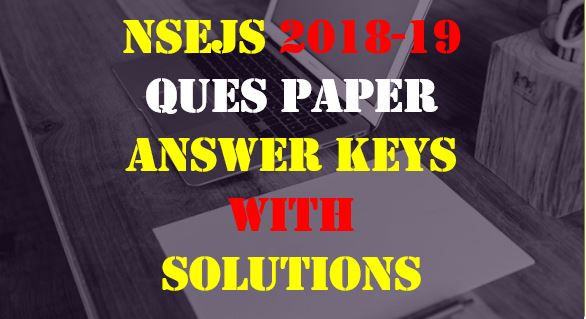# NSEJS 2018-19 Question Paper With Answer Keys And Solutions

Hi students, welcome to AmansMathsBlogs (AMB). On this article, you will get NSEJS 2018-19 Question Paper With Answer Keys And Solutions.

NSEJS 2018-19 is an IJSO Stage 1 exam that is conducted by IAPT (Indian Association of Physics Teacher). In 2018-19 session, NSEJS is conducted on 18 November 2018 (Sunday).

## NSEJS Maths Questions Bank EBook

Get NSEJS 2008 to 2018 Maths Questions Bank with Solutions. To get this combo ebook, click below RED button.These questions are solved by me, AMAN RAJ, I am maths faculty of IIT Maths Foundation course and founder of this website AmansMathsBlogs (AMB).

NSEJS
Previous Year Question Papers
2008-092009-102010-112011-122012-13
2013-142014-152015-162016-172017-18
2018-192019-20

## NSEJS 2018-19 Question Paper Code JS511

Read More : 129 Maths Short Tricks

Ques NoAns KeyQues NoAns KeyQues NoAns KeyQues NoAns Key
1B6C11B16B
2A7B12B17B
3D8B13B18B
4C9C14A19B
5A10A15C20C
Ques NoAns KeyQues NoAns KeyQues NoAns KeyQues NoAns Key
21D26D31A36C
22B27C32A37D
23C28D33A38A
24A29B34A39C
25C30B35A40C
Ques NoAns KeyQues NoAns KeyQues NoAns KeyQues NoAns Key
41C46C, D51A56B
42D47NA52C57NA
43D48B53C58A
44C, D49B54A59D
45A50B55B60D
Ques NoAns KeyQues NoAns KeyQues NoAns KeyQues NoAns Key
61A66D71B76B
62B67C72A77D
63B68D73C78B
64A69C74C79A
65D70A75A80A

## NSEJS 2018-19 Solutions MATHS

NSEJS 2018-19 Maths Questions Bank With Solutions: Ques No 41

Let AB be a diameter of a circle C1 of radius 30 cm and with centre O. Two circles C2 and C3 of radii 15 cm and 10 cm touch internally at A and B respectively. A fourth circle C4 touches C1, C2 and C3. What is the largest possible radius of C4?

Options:

A. 12 cm

B. 15 cm

C. 20 cm

D. 30 cm

Solution:NSEJS 2018-19 Maths Questions Bank With Solutions: Ques No 42

A 5×5×5 cube is built using unit cubes. How many different cuboids (that differ in at least one-unit cube) can be formed using the same number of unit cubes?

Options:

A. 1000

B. 1728

C. 2730

D. 3375

Solution: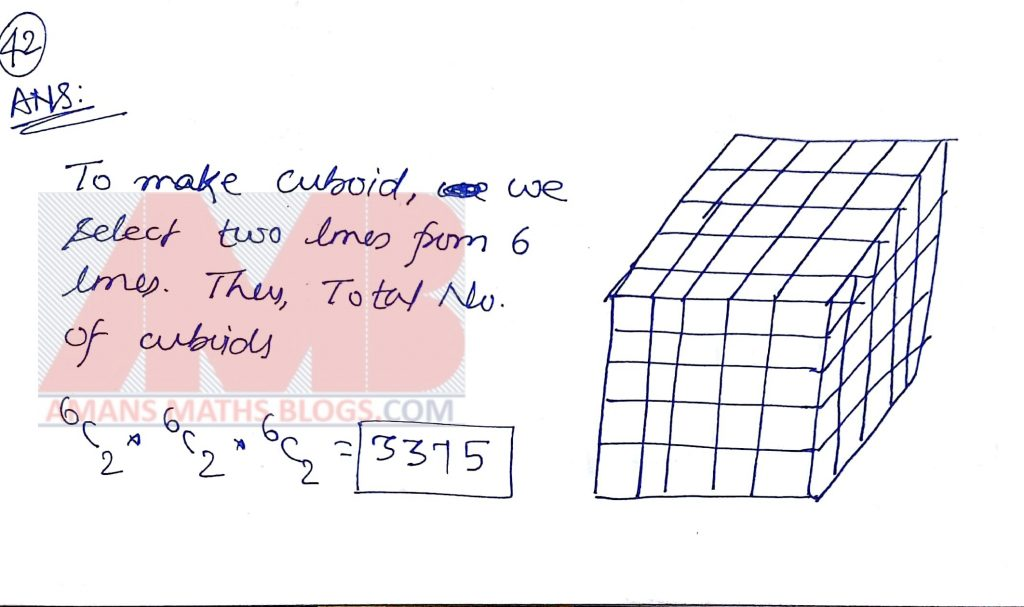NSEJS 2018-19 Maths Questions Bank With Solutions: Ques No 43

What is the largest value of the positive integer k such that k divides n2(n2 – 1)(n2 – n – 2) for every natural number n?

Options:

A. 6

B. 12

C. 24

D. 48

Solution: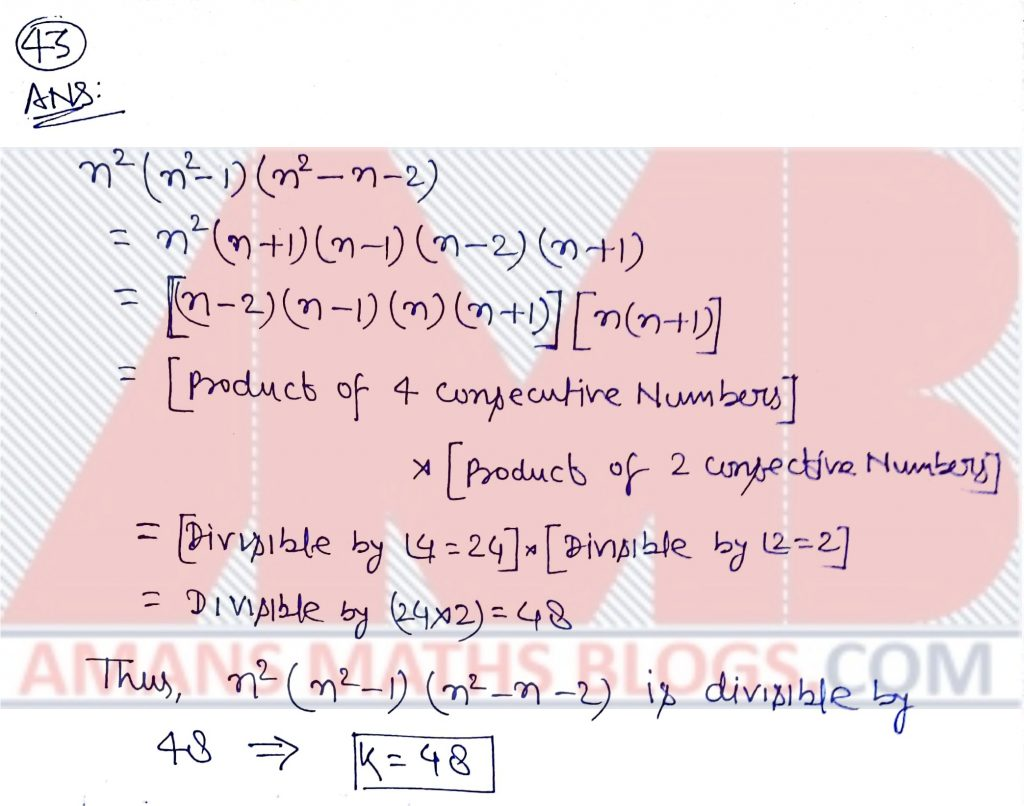NSEJS 2018-19 Maths Questions Bank With Solutions: Ques No 44

A person kept rolling a regular (six faced) die until one of the numbers appeared third time on the top. This happened in 12th throw and the sum of all the numbers in 12 throws was 46. Which number appeared least number of times?

Options:

A. 6

B. 4

C. 2

D. 1

Solution: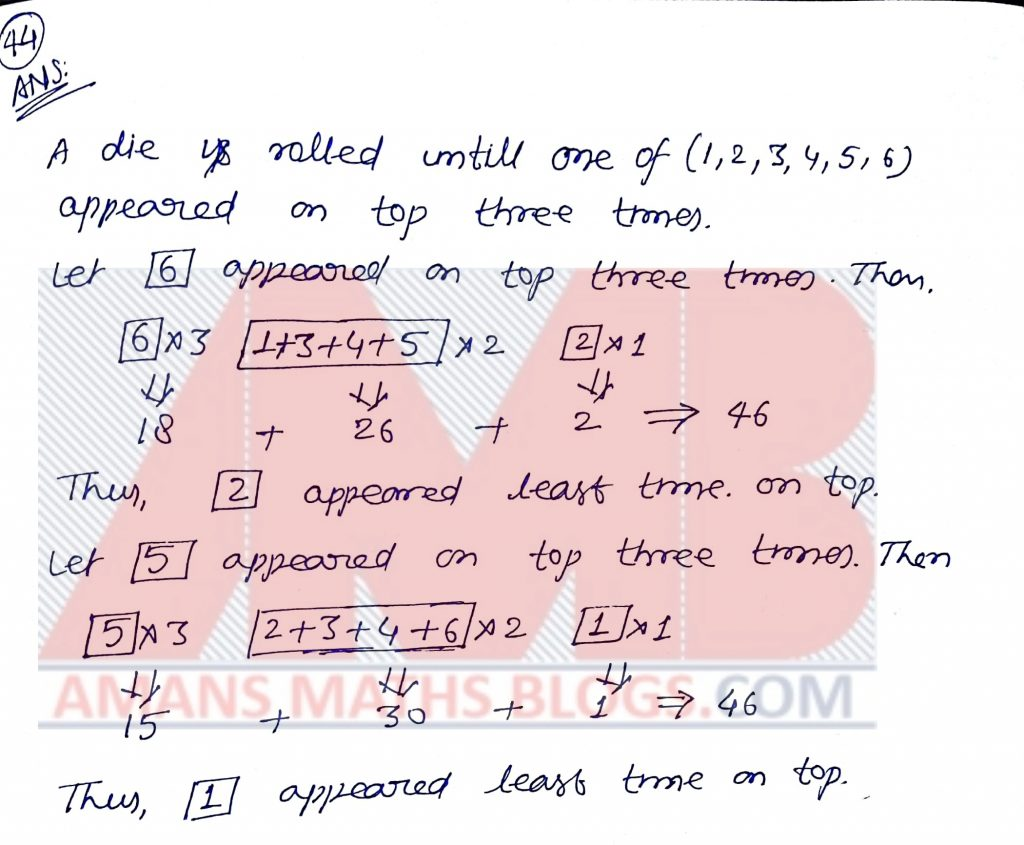NSEJS 2018-19 Maths Questions Bank With Solutions: Ques No 45

In a square ABCD, a point P is inside the square such that ABP is an equilateral triangle. The segment AP cuts the diagonal BD in E. Suppose AE = 2. The area of ABCD is

Options:

A. 4 + 2Root(3)

B. 5 + 2Root(3)

C. 4 + 4Root(3)

D. 5 + 4Root(3)

Solution: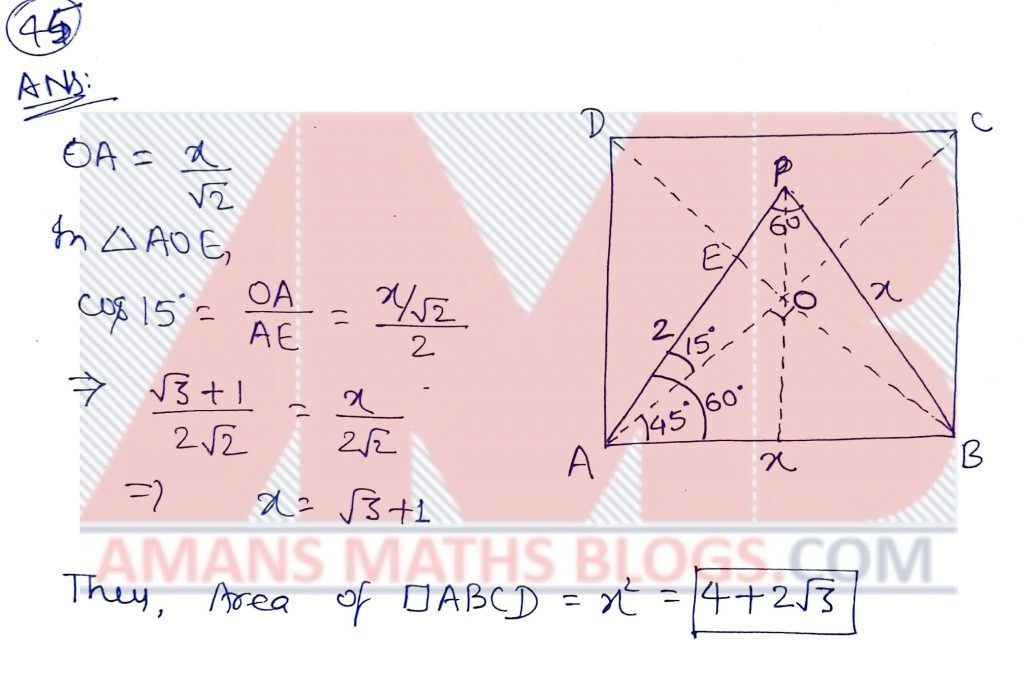NSEJS 2018-19 Maths Questions Bank With Solutions: Ques No 46

Let n be a positive integer not divisible by 6. Suppose n has positive divisors. The number of positive divisors of 9n is

Options:

A. 54

B. 36

C. 18

D. 12

Solution: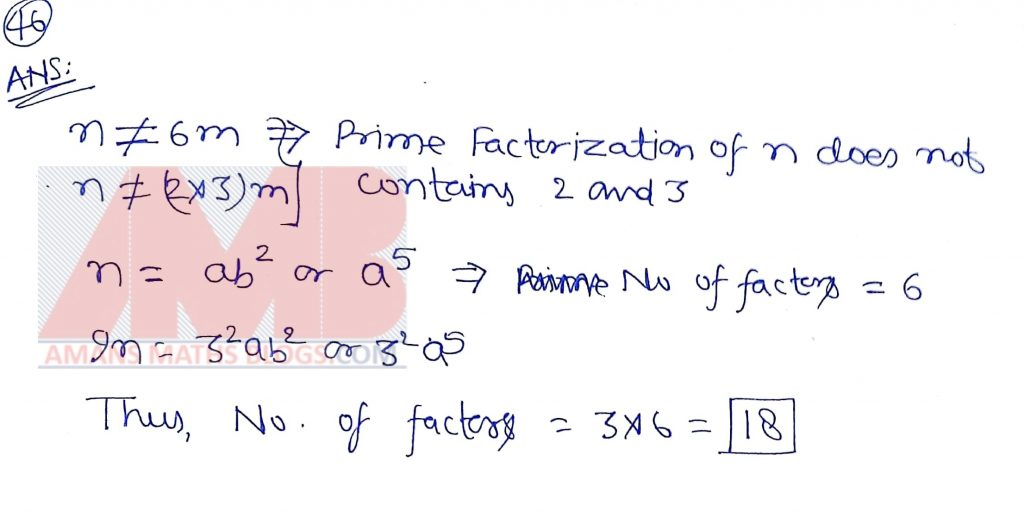NSEJS 2018-19 Maths Questions Bank With Solutions: Ques No 47

The value of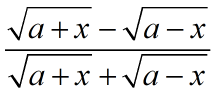, when x = 2ab/(b2 + 1) is:

Options:

A. a

B. b

C. x

D. 0

Answer Key: BONUS as wrong print in x = 2a/(b2 + 1)

Solution:NSEJS 2018-19 Maths Questions Bank With Solutions: Ques No 48

Two regular polygons of different number of sides are taken. In one of them, its sides are colored red and diagonals are colored green. In other, the sides are colored green and diagonals are colored red. Suppose there are 103 red lines and 80 green lines. The total number of sides the two polygons together have is:

Options:

A. 23

B. 28

C. 33

D. 38

Solution: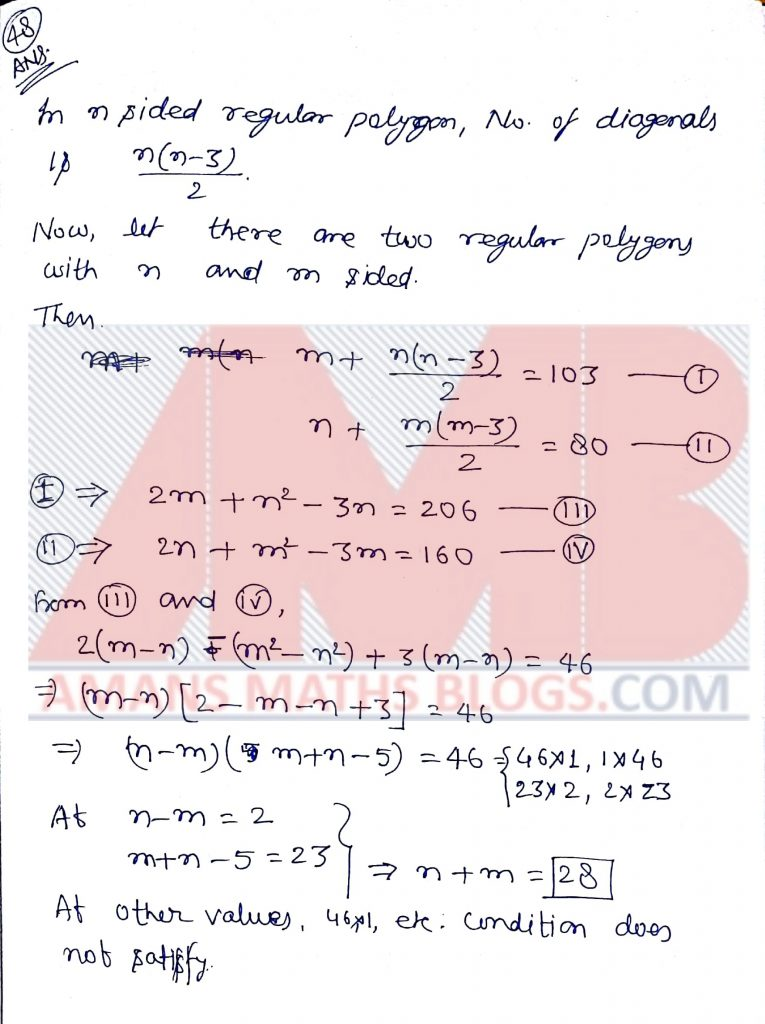NSEJS 2018-19 Maths Questions Bank With Solutions: Ques No 49

A box contains some red and some yellow balls. If one red ball is removed, one seventh of the remaining balls would be red. If one yellow ball is removed, one-sixth of the remaining balls would be red. If n denotes the total number of balls in the box, then the sum of the digits of n is:

Options:

A. 6

B. 7

C. 8

D. 9

NSEJS 2018-19 Maths Questions Bank With Solutions: Ques No 50

Let ABCD be a rectangle. Let X and Y be points respectively on AB and CD such that AX:XB = 1:2 = CY:YD. Join AY and CX. Let BY intersect CX in K. Let DX intersect AY in L. If m/n denotes the ratio of the area of XKYL to that of the ABCD, then (m + n) is

Options:

A. 9

B. 11

C. 13

D. 15

Solution:NSEJS 2018-19 Maths Questions Bank With Solutions: Ques No 51

Let ABC be an equilateral triangle. The bisector of ∠BAC meets the circumcircle of ABC in D. Suppose DB + DC = 4. The diameter of the circumcircle of ABC is

Options:

A. 4

B. 3Root(3)

C. 2Root(3)

D. 2

Solution: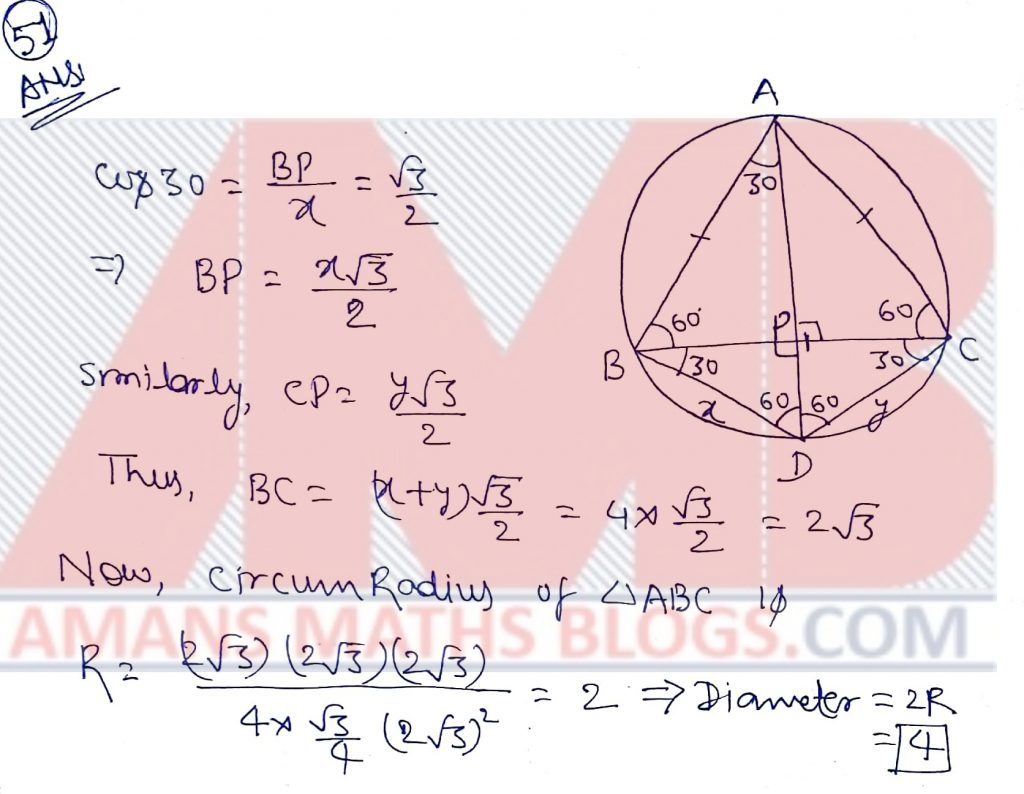NSEJS 2018-19 Maths Questions Bank With Solutions: Ques No 52

Let Tk denote the kth term of an arithmetic progression. Suppose there are positive integers m ≠ n such that Tm = 1/n and Tn = 1/m. Then Tmn is

Options:

A. 1/mn

B. 1/m + 1/n

C. 1

D. 0

Solution:NSEJS 2018-19 Maths Questions Bank With Solutions: Ques No 53

In a triangle ABC, let AD be the median from A. Let E be a point on AD such that AE:ED = 1:2 and let BE extended meets AC in F. The ratio of AF/FC is

Options:

A. 1/6

B. 1/5

C. 1/4

D. 1/3

Solution:NSEJS 2018-19 Maths Questions Bank With Solutions: Ques No 54

If sinθ and cosθ are roots of the equation px2 + qx + r = 0, then

Options:

A. p2 – q2 + 2pr = 0

B. (p + r)2 = q2 – r2

C. p2 + q2 – 2pr = 0

D. (p – r)2 = q2 + r2

Solution:NSEJS 2018-19 Maths Questions Bank With Solutions: Ques No 55

For a regular k-sided polygon, let a(k) denotes its interior angle. Suppose n > 4 is such that a(n – 2), a(n), a(n + 3) forms an arithmetic progression. The sum of digits of n is

Options:

A. 2

B. 3

C. 4

D. 5

Solution: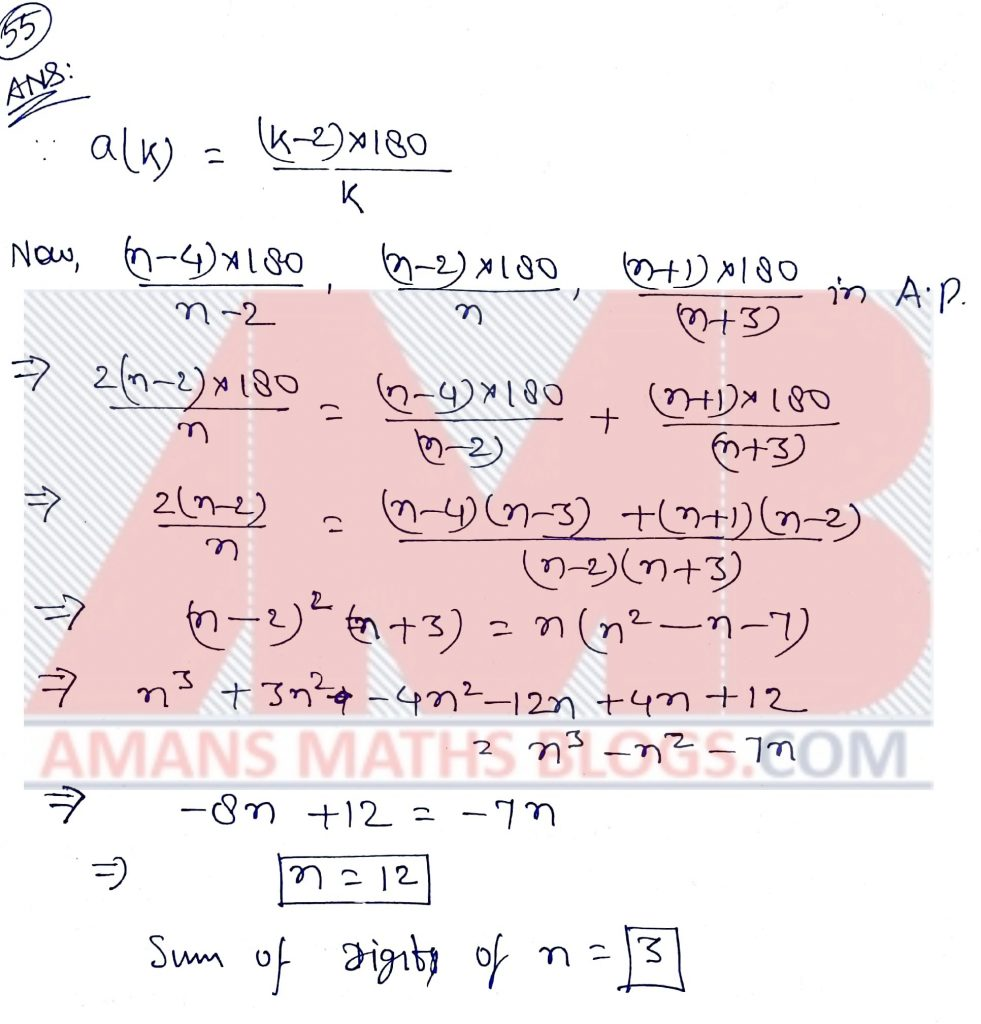NSEJS 2018-19 Maths Questions Bank With Solutions: Ques No 56

The sum of 5 numbers in geometric progression is 24. The sum of their reciprocals is 6. The product of the terms of the geometric progression is

Options:

A. 36

B. 32

C. 24

D. 18

Solution:NSEJS 2018-19 Maths Questions Bank With Solutions: Ques No 57

Digits a and b are such that the product 4a1 and 25b is divisible by 36 (in base 10). The number of ordered pairs (a, b) is

Options:

A. 15

B. 8

C. 6

D. 4

Solution: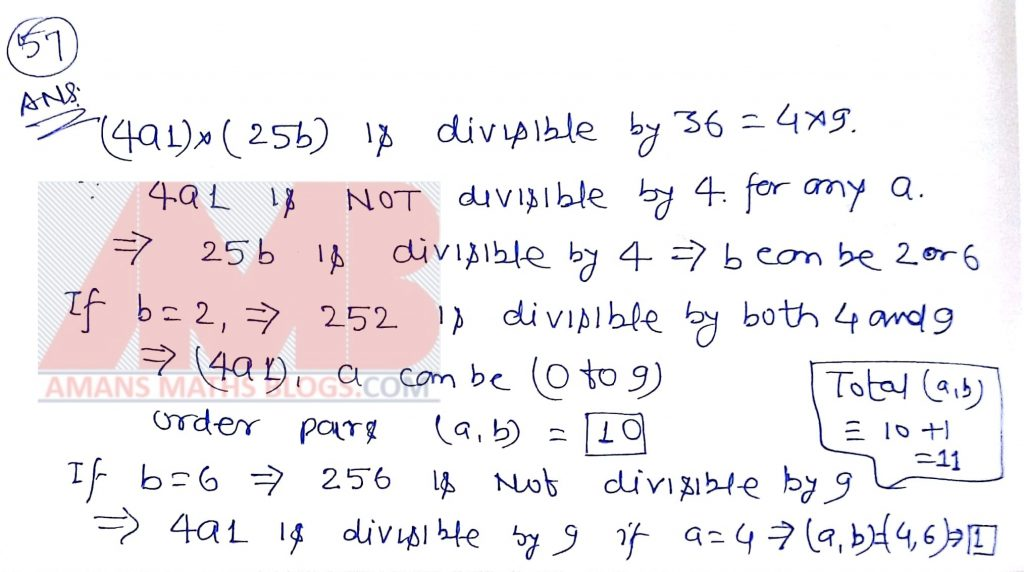NSEJS 2018-19 Maths Questions Bank With Solutions: Ques No 58

The integer closest to, where there are 2018 ones and 1009 twos, is

Options:

A. (101009 – 1)/3

B. (101009 – 1)/9

C. (102018 – 1)/3

D. (102018 – 1)/9

Solution: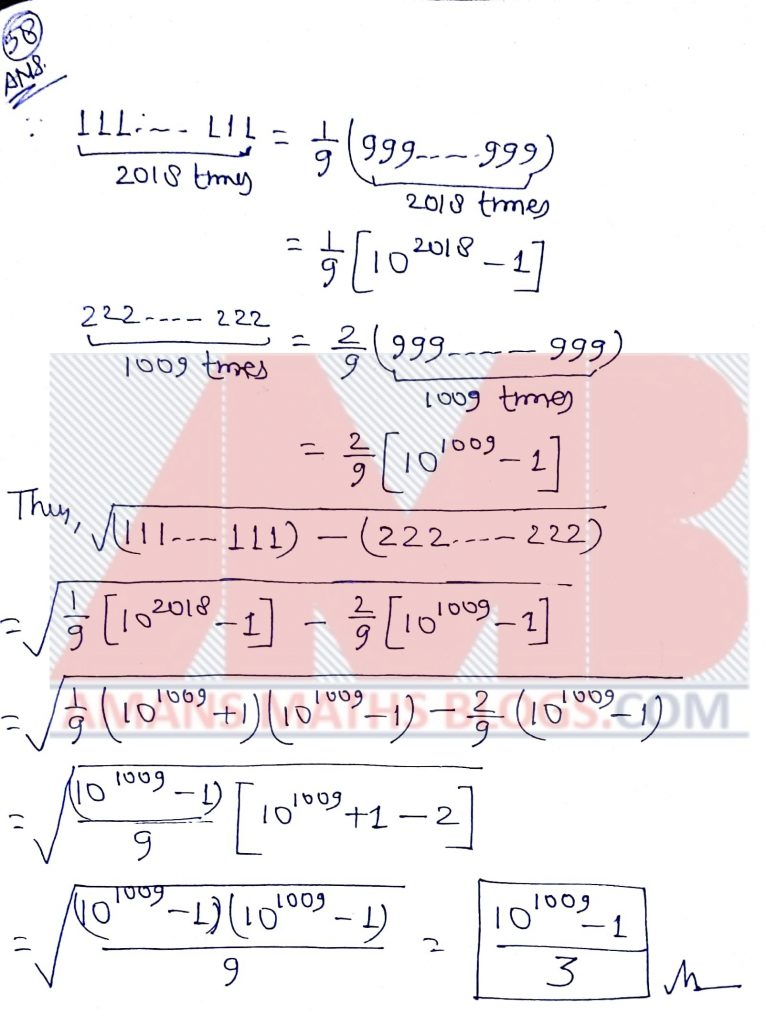NSEJS 2018-19 Maths Questions Bank With Solutions: Ques No 59

In a triangle ABC, a point D on AB is such that AD:AB = 1:4 and DE is parallel to BC with E on AC. Let M and N be the mid-points of DE and BC respectively. What is the ratio of the area of the quadrilateral BNMD to that of the triangle ABC?

Options:

A. 1/4

B. 9/32

C. 7/32

D. 15/32

Solution: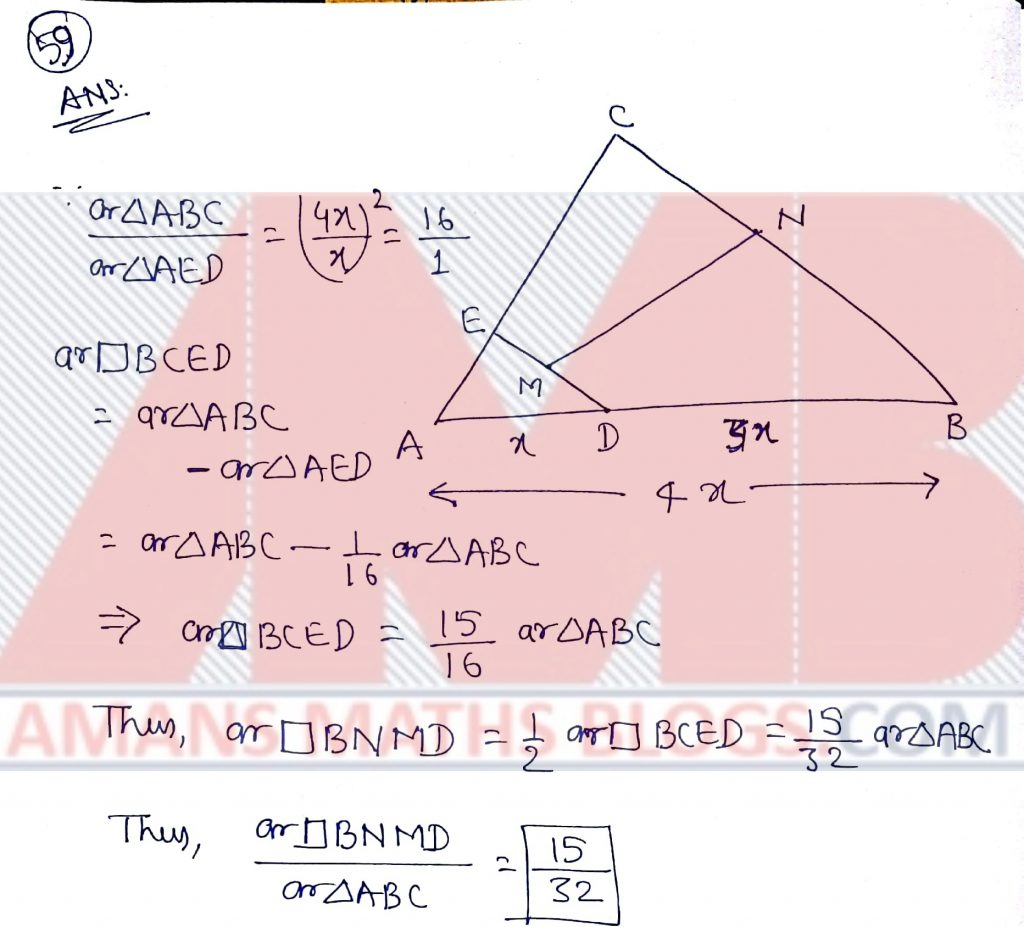NSEJS 2018-19 Maths Questions Bank With Solutions: Ques No 60

The number of distinct integers in the collection [102/1], [102/2], [102/3], [102/4], …, [102/20]

Options:

A. 20

B. 18

C. 17

D. 15

Solution: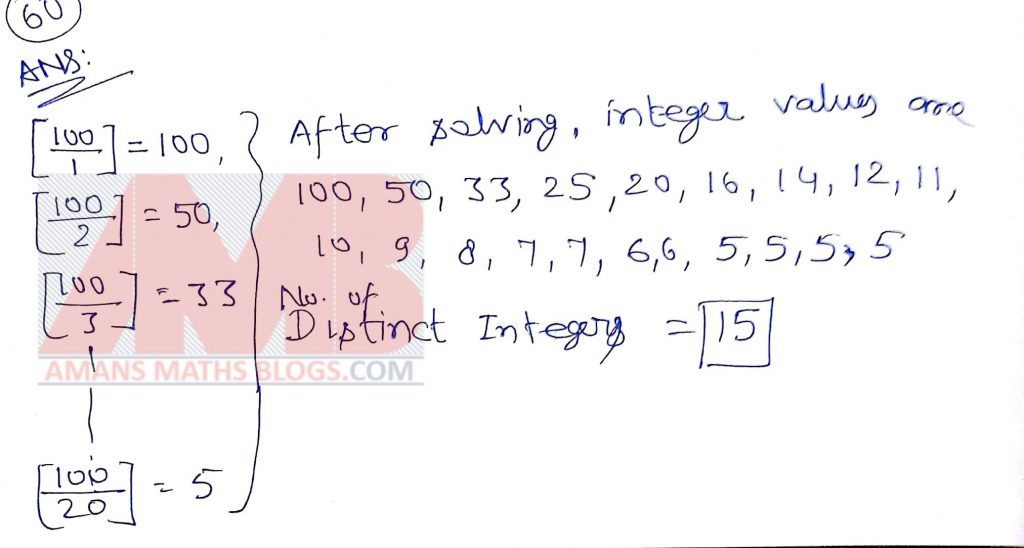NSEJS
Previous Year Question Papers
2008-092009-102010-112011-122012-13
2013-142014-152015-162016-172017-18
2018-192019-20

## NSEJS Maths Questions Bank EBook

Get NSEJS 2008 to 2018 Maths Questions Bank with Solutions. To get this combo ebook, click below RED button.AMBiPi
error: Content is protected !!